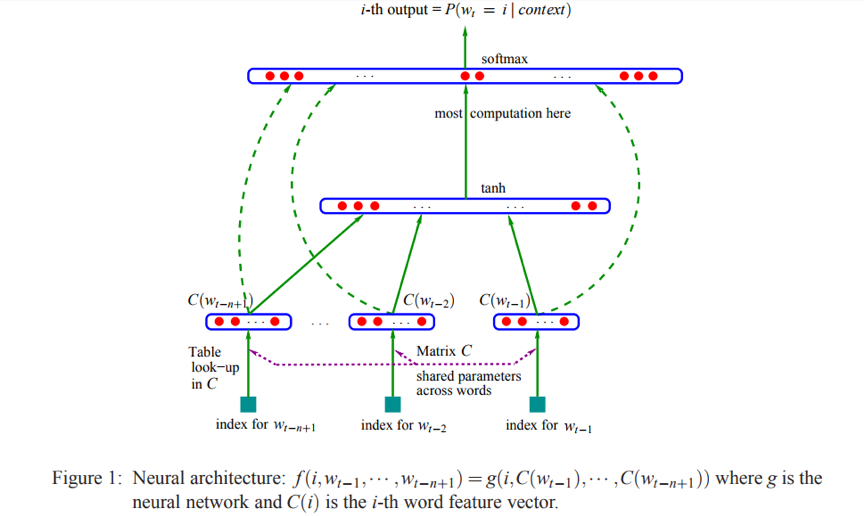# 词向量的前世今生

Published:

1. 使用训练出的词向量作为输入特征，提升现有系统，如应用在情感分析、词性标注、语言翻译等神经网络中的输入层。
2. 直接从语言学的角度对词向量进行应用，如使用向量的距离表示词语相似度、query相关性等。

## Why Word Embedding?## 前世

Word2Vec算法使Distributed representation真正受到学术界、工业界的认可，它属于predictive方法，传承自Bengio的”Neural Probabilistic Language Models”。NNLM是Distributed representation for words早期的重要工作，Word2Vec的很多方面受到该篇论文启发。文章提出了一个3层的网络结构(embedding输入层，tanh隐层，softmax输出层)，通过最大化词序列的联合概率来学习word embedding。

### 背景知识

NNLM的目标函数是给定上文时下一个词的条件概率，该目标函数源于统计语言模型(statistical model of language)。一般地，统计语言模型将一个句子(由多个词构成的序列)的出现概率建模为：

$\widehat{P}(w_{1}^{T})= \prod_{t=1}^{T}\widehat{P}(w_{t}|w_{1}^{t-1})$

$\widehat{P}(w_{t}|w_{1}^{t-1})\approx \widehat{P}(w_{t}|w_{t-n+1}^{t-1})$

$\widehat{P}(w|Context(w))=F(w,Context(w),\theta)$

$L(\theta)=\sum_{w\in C}log p(w|Context(w))$

### NNLM上图是NNLM的网络结构图，输入是target word的context(前n个词在矩阵C中的index，等价于在vocabulary中的index)，输出是一个长度为$V$的向量，其中第i维代表target word为$i$ ($i$同样是词在vocabulary中的index)在当前context下的条件概率。矩阵$C$是NNLM的关键部分，论文中原文是：
 In practice, $C$ is represented by a $V$ * m matrix of free parameters. $C$ being shared across all the words in the context.
 实际上，$C$矩阵存储的就是语料中所有词的词向量，它的行数$V$是语料中词的个数，列数m为词向量的长度。上图$g$代表的是神经网络的映射(mapping)，由图可知它包括了tanh层和softmax层。NNLM的目标函数为：
$\widehat{P}(w_{t}|w_{t-n+1}^{t-1})=\frac{e^{y_{w_{t}}}}{\sum_{i}e^{y_{i}}},\ where\ y=b+Wx+Utanh(d+Hx)$

$|V|(1 + nm + h) + h(1 + (n - 1)m)=O(N(nm+h))$

## 今生

2013年word2vec横空出世，背后的原理在Mikolov的两篇论文”Efficient Estimation of Word Representations in VectorSpace”, “Distributed Representations of Words and Phrases and their Compositionality”中有详细的介绍。CBOW的目标函数是：

$\widehat{P}(w_{t}|w_{c})=\frac{e^{v_{w_{t}}^{T}v_{w_{c}}}}{\sum_{w=1}^{N}{v_{w}^{T}v_{w_{c}}}},\ where\ v_{w_{c}}=\sum_{i\in context(w_{t})}C(w_{i})$

$L(\theta)=-\frac{1}{T}\sum_{t}log\ \widehat P(w_{t}|w_{c})+R(\theta)$

$nm+mlog_{2}(N)=O(mlog_{2}(N))$

Skip-gram的目标函数是：

$\prod_{-c\leq j\leq c, j\ne t}\widehat{P}(w_{j}|w_{t})=\frac{e^{v_{w_{j}}^{T}v_{w_{t}}}}{\sum_{w=1}^{N}{v_{w}^{T}v_{w_{t}}}}$

$L(\theta)=-\frac{1}{T}\sum_{t}\sum_{-c\leq j\leq c, j\ne t}log\ \widehat{P}(w_{j}|w_{t})+R(\theta)$

$n(m+nlog_{2}(N))=O(nmlog_{2}(N))$

### 结论

NNLM到word2vec的发展使得计算复杂度由O(N)的线性级降低到对数级

## 参考文献

 Bengio Y, Schwenk H, Senécal J S, et al. Neural Probabilistic Language Models[J]. Journal of Machine Learning Research, 2003, 3(6):1137-1155.
 Mikolov T, Chen K, Corrado G, et al. Efficient Estimation of Word Representations in Vector Space[J]. Computer Science, 2013.
 Mikolov T, Sutskever I, Chen K, et al. Distributed representations of words and phrases and their compositionality[C]// International Conference on Neural Information Processing Systems. Curran Associates Inc. 2013:3111-3119.

Tags: